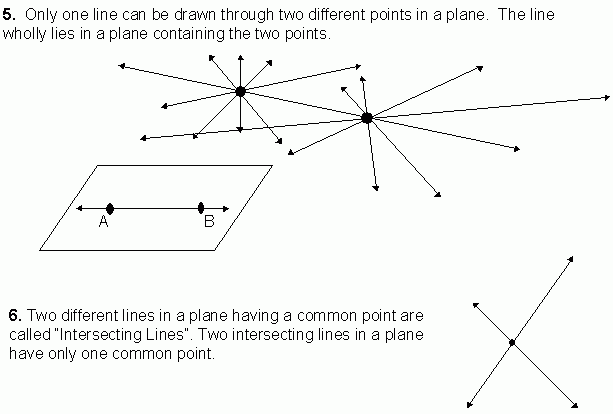Email us to get an instant 20% discount on highly effective K-12 Math & English kwizNET Programs!

#### Online Quiz (WorksheetABCD)

Questions Per Quiz = 2 4 6 8 10

### Middle/High School Algebra, Geometry, and Statistics (AGS)5.2 Basic Geometrical Concepts - Parallel Lines, Collinear Points and PlaneFor videos and additional resources on this topic, please see: MyndBook.com/go.php?to=207_885 Directions: Answer the following questions. Also on a sheet of paper draw a collinear points, non-collinear points and parallel and intersecting lines.
 Q 1: The flat surface of a white board gives an idea of a _____.lineplanepoint Q 2: Number of lines that can be drawn through collinear points is _____.twooneinfinity Q 3: If C is not a point on the line AB, then A, B, C are called _____ points.linearcollinearnon-collinear Q 4: 'A' and 'B' are two points on a paper. If a line is passing through 'A' and 'B', then the number of other lines that can be drawn through 'A' and 'B' is _____.manytwoonezero Q 5: The surface of a box, the cover page of a book etc., are examples of parts of _____.linesplanespoints Q 6: A plane is a flat surface extending _____ in all direction.indefinitelyfinitelydefinitely Q 7: A, B, C and D are four points such that not any three of them are on the same line. Then number of lines that can be drawn through them taking two points at a time is _____.sixfourtwo Q 8: Number of lines that can be drawn through three non-collinear points, taking two points at a time is _____.twothreeone Question 9: This question is available to subscribers only! Question 10: This question is available to subscribers only!